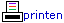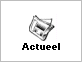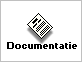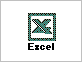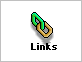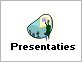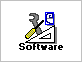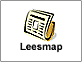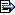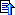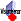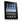## 1. Technieken algemeen

There are many techniques that can be used to solve sudoku puzzles. Listed below are the techniques that the sudoku solver uses in order of difficulty. The Glossary explains the various terms used in describing techniques.

Naked Single
If you can eliminate all candidates for a cell except one, the cell must contain the remaining value.

Hidden Single
The Hidden Single technique is based on the fact that all units (rows, columns, and blocks) must contain each digit 1-9. If a candidate can only exist in one cell in a unit, the cell must contain that digit.

Block Line
If a value can exist in only one row or column within a block, then the value cannot lie anywhere else in that row or column outside of that block.

Double Block Line
Double Block Line is similar to Block Line except... doubled! With this technique, two blocks and two lines are taken into account.

Naked Subset
If there are N cells in one unit that contain N candidates between them, then the candidates can be removed from all the other cells in the unit.

Hidden Subset
If in a unit, there is a set of N candidates that only appears in N cells, then you can remove all other candidates from those cells.

X-Wing
If two rows have a candidate in exactly two cells that lie on the same two columns, then the candidate can be eliminated from all other cells in the those columns.

Swordfish
This technique is similar to the X-Wing technique, except it looks at three rows and columns. If three rows have a candidate in up to three cells that lie on the same three columns, then the candidate can be eliminated from all other cells in the those columns.

Jellyfish
This technique is similar to the Swordfish technique, except it looks at four rows and columns. If four rows have a candidate in up to four cells that lie on the same four columns, then the candidate can be eliminated from all other cells in the those columns.

XY-Wing
Look for a cell with exactly two candidates, XY. If there are two cells, XZ and YZ, that both share a unit with XY, then we can be certain that either XZ or YZ will contain the value Z. So, we can remove the candidate Z from the intersection of XZ and YZ.

XYZ-Wing
This technique is very similar to the XY-Wing technique. Find a cell with candidates XYZ that has two buddies, XZ and YZ. The candidate Z can be eliminated from the intersection of XYZ, XZ, and YZ.

Colouring
The Colouring technique is based on the idea of conjugates; If there are only two cells in a unit with a candidate digit, one cell must contain the digit and the other must not. Find a conjugate pair and colour one cell red, and the other green. Then find any conjugates for those two cells, and alternate the colour of each conjugate. Repeat until there are no conjugates left. The candidate digit must be in either all the red cells or all the green cells. If it would be impossible for the digit to be in one colour, it must be in the other.

XY-Chain
The XY-Chain technique is an extension of the XY-Wing technique where a chain of cells is formed. Each link in the chain is a pair of cells in the same unit that have two candidates each and share a common candidate. If you form a chain of links, and the unused candidates at the endpoints of the chain are the same, you can remove that candidate from the intersection of the endpoints.